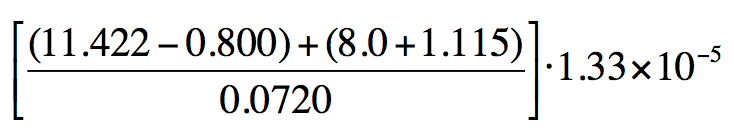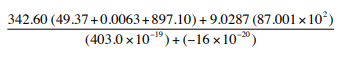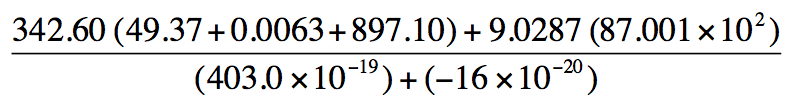Ch.3 - Experimental ErrorWorksheetSee all chapters
 Ch.1 - Chemical Measurements 2hrs & 8mins 0% complete Worksheet Ch.2 - Tools of the Trade 1hr & 26mins 0% complete Worksheet Ch.3 - Experimental Error 1hr & 53mins 0% complete Worksheet Ch.4 + 5 - Statistics, Quality Assurance and Calibration Methods 2hrs & 1min 0% complete Worksheet Ch.6 - Chemical Equilibrium 3hrs & 43mins 0% complete Worksheet Ch.7 - Activity and the Systematic Treatment of Equilibrium 1hr & 1min 0% complete Worksheet Ch.8 - Monoprotic Acid-Base Equilibria 1hr & 52mins 0% complete Worksheet Ch.9 - Polyprotic Acid-Base Equilibria 2hrs & 16mins 0% complete Worksheet Ch.10 - Acid-Base Titrations 2hrs & 40mins 0% complete Worksheet Ch.11 - EDTA Titrations 1hr & 37mins 0% complete Worksheet Ch.12 - Advanced Topics in Equilibrium 1hr & 18mins 0% complete Worksheet Ch.13 - Fundamentals of Electrochemistry 2hrs & 27mins 0% complete Worksheet Ch.14 - Electrodes and Potentiometry 43mins 0% complete Worksheet Ch.15 - Redox Titrations 1hr & 14mins 0% complete Worksheet Ch.16 - Electroanalytical Techniques 1hr & 3mins 0% complete Worksheet Ch.17 - Fundamentals of Spectrophotometry 55mins 0% complete Worksheet BONUS: Chemical Kinetics Not available yet

# Multiplication and Division Operations

See all sections

Multiplication and division of values written in scientific notation.

###### Multiplication and Division

When multiplying or dividing your final answer will have the least number of significant figures.

Concept #1: Multiplication and Division Operations

Example #1: Using the method discussed above, determine the answer when the following values are multiplied.

(2.134 x 105) ● (1.6 x 10-3) ● (3.07 x 106

Example #2: Using the methods discussed above, determine the answer for the following mixed operations question.

[(7.33 x 108) ● (9.89 x 10-1)] / (6.12 x 1011)

###### Multiplication and Division Calculations

Example #3: Perform the following calculation to the right number of sig figs:

[(9.12 x 10-5) + (6.33 x 10-3)] / [(1.15 x 107) - (3.72 x 106)]

Practice: Perform the following calculation for the image below to the right number of sig figs:

[(11.422-0.800) + (8.0 + 1.115)/0.0720] 1.33 x 10-5Practice: Compute the following and determine the correct number of significant figures in the answer for the image below: